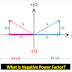The power factor of an Electrical system represents how much part of the main power supply applied to the load is used to do the useful work and how much part is wasting. Generally, the value of the power factor varies between 0 to 1. It may be lagging or leading in nature.

Sometimes the value of the power factor goes below Zero(0) or in negative value which is called Negative Power Factor. In this article, we are going to know what is the causes of the negative power factor, its effect, and how to improve it.

## Negative Power Factor

The cosine of the angle between voltage and current when electrical power is flowing in reverse direction or opposite direction is called the Negative Power Factor.

Generally, a negative Power Factor occurs when power flows from load to source.
The negative power factor mainly indicates to leading power factor from the point of view of the main power source.
The negative power factor happens with the capacitor, inductor, transformer, motors, etc.

## Causes of Negative Power Factor

So, a negative power factor occurs when electrical power generated from the load and flows back towards the source. For example, an induction motor is used in a railway locomotive. When that train going from upside to downside of a hill, it will generate electrical power and power will flow towards the main Power source. In that time Negative Power Factor occurs.

Another reason for occurring negative power factor is when in an electrical circuit both inductive and capacitive load is connected. In this case, if the reactive part of the consuming power is more capacitive than inductive.

## Effects of Negative Power Factor

Negative Power Factor causes the terminal voltage across the load to rise above its open circuit value. This may damage the voltage-sensitive load.

Negative power factor can damage the power generating devices such as solar panels, generators, etc.

## Negative Power Factor Improvement

As there are two reasons for the occurring negative power factor, there also two methods for improvement.

If the negative power factor occurs due to power flow in the opposite direction, then it should be stopped. A PN Junction diode can be used to stop power flow in reverse direction.

Another way to improve the negative power factor is, controlling reactive power. If the power factor is lagging then it is to be added to the load to compensate for the power factor. If the power factor is leading then reactive power is to absorbed.

Thank you for visiting the website. keep visiting for more updates.

Negative Power Factor Causes, Effects, ImprovementReviewed by manoranjan das on 11:29 am Rating: 5